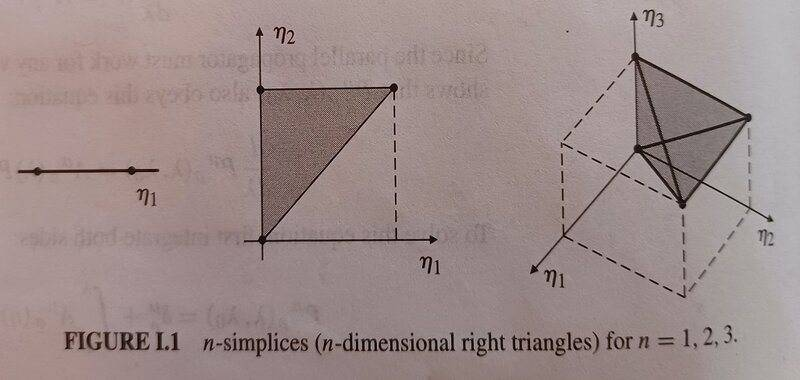# How do a bunch of integrals make an n-simplex or an n-cube?

Gold Member
TL;DR Summary
Problem with series of integrals in parallel propagator. Parallel transporting vectors.
This question arises from Carroll's Appendix I on the parallel propagator where he shows that, in matrix notation, it is given by$$P\left(\lambda,\lambda_0\right)=I+\sum_{n=1}^{n=\infty}T_n$$and$$T_n=\int_{\lambda_0}^{\lambda}{\int_{\lambda_0}^{\eta_n}\int_{\lambda_0}^{\eta_{n-1}}{\ldots\int_{\lambda_0}^{\eta_2}A\left(\eta_n\right)A\left(\eta_{n-1}\right)\ldots A\left(\eta_1\right)}d^n\eta}$$$$\frac{1}{n!}\int_{\lambda_0}^{\lambda}{\int_{\lambda_0}^{\lambda}\int_{\lambda_0}^{\lambda}{\ldots\int_{\lambda_0}^{\lambda}\mathcal{P}\left[A\left(\eta_n\right)A\left(\eta_{n-1}\right)\ldots A\left(\eta_1\right)\right]}d^n\eta}$$where ##\mathcal{P}## orders the matrices ##A\left(\eta_i\right)## so that ##\eta_n\geq\eta_{n-1}\geq\ldots\geq\eta_1##.

Carroll says that the ##n##th term in the first series of integrals is an integral over an ##n##-dimensional right triangle or ##n##-simplex:$$T_1=\int_{\lambda_0}^{\lambda}{A\left(\eta_1\right)d\eta_1}$$$$T_2=\int_{\lambda_0}^{\lambda}{\int_{\lambda_0}^{\eta_2}A\left(\eta_2\right)A\left(\eta_1\right)d\eta_1d\eta_2}$$$$T_2=\int_{\lambda_0}^{\lambda}{\int_{\lambda_0}^{\eta_3}\int_{\lambda_0}^{\eta_2}A\left(\eta_3\right)A\left(\eta_2\right)A\left(\eta_1\right)d^3\eta}$$and illustrates them thus:I can see how a 2-simplex with short sides equal is half a square and how that carries on for more dimensions but I can't see how the expression, for example, for ##T_2## is an integral over a right angle triangle, let alone one with short sides equal ##\lambda-\lambda_0##.

It would seem that what exactly ##A## is is immaterial. I tested calculating ##T_2## both ways for three cases$$A\left(x\right)=3x^2,A_{\ \ \rho}^\mu\left(\lambda\right)=\left(\begin{matrix}0&\sin{\psi}\cos{\psi}\\-\cot{\psi}&0\\\end{matrix}\right),\ A_{\ \ \rho}^\mu\left(x\right)=\left(\begin{matrix}2x&3x^2\\4x^3&5x^4\\\end{matrix}\right)$$the first two gave the same answer by both methods and the third did not (I may easily have made a mistake on that.) The second is what ##A_{\ \ \rho}^\mu## would be for propagating a vector on the surface of a sphere at constant latitude ##\psi##. It was much easier to use the second method (over an ##n##-cube) and that did indeed parallel propagate vectors round lines of constant latitude very well.

If anybody can help me understand why the integrals are integrals over ##n##-simplices or ##n##-cubes and how ##\mathcal{P}## orders the matrices ##A\left(\eta_i\right)## so that ##\eta_n\geq\eta_{n-1}\geq\ldots\geq\eta_1## (the ##\eta_i## seem to be moving targets) I would greatly appreciate it.

•